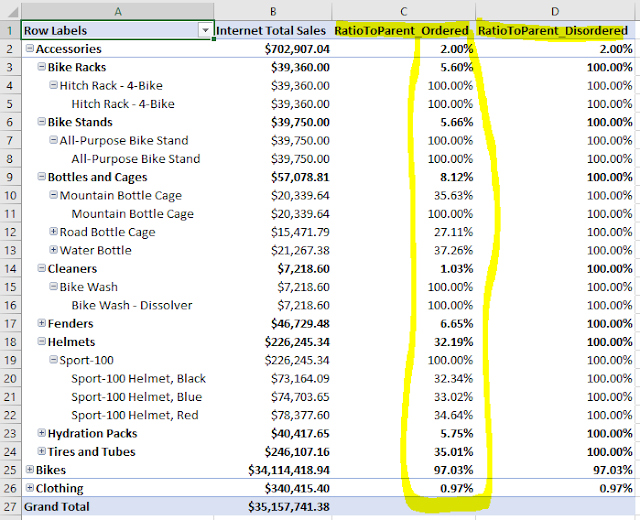Log in  ::  Register  ::  Not logged in

# DAX calculations with hierarchies: Set the order straight.

[2017-Apr-30] If you have ever tried to create calculated measures in SSAS Tabular/ Power BI models where different levels of your model hierarchy had to be considered then you'd understand how DAX language doesn't have a straight way to identify parent & child levels in order to compute necessary metric results. There is a good article http://www.sqlbi.com/articles/clever-hierarchy-handling-in-dax/ by Alberto Ferrari where he explains a workaround approach to handle hierarchies in DAX.

I made one step further, based on my recent client project experience, and created a new example with one extra level for the Product dimension using Adventure Works Internet Sales tabular database. Here is the code to calculate Sales Ratio to a Parent level for all levels (Category, Sub Category, Model and Product Name)

`RatioToParent:=IF (    ISFILTERED ( Product[HCategory] ),    SUM ( 'Internet Sales'[Sales Amount] )        / CALCULATE ( SUM ( 'Internet Sales'[Sales Amount] ), ALL ( Product[HCategory] ) ),    IF (        ISFILTERED ( Product[HSubcategory] ),        SUM ( 'Internet Sales'[Sales Amount] )            / CALCULATE ( SUM ( 'Internet Sales'[Sales Amount] ), ALL ( Product[HSubcategory] ) ),        IF (            ISFILTERED ( Product[HModel] ),            SUM ( 'Internet Sales'[Sales Amount] )                / CALCULATE (                    SUM ( 'Internet Sales'[Sales Amount] ),                    ALL ( Product[HModel] )                ),            IF (                ISFILTERED ( Product[HProduct] ),                SUM ( 'Internet Sales'[Sales Amount] )                    / CALCULATE ( SUM ( 'Internet Sales'[Sales Amount] ), ALL ( Product[HProduct] ) )            )        )    ))`

However, when I looked at the results for this new RatioToParent measure using Excel, I saw some weird values: only the to Category level showed me correct calculations and all other Product hierarchy levels had 100%.Then I changed the order of levels in my DAX calculated measure from (Category > Sub Category > Model > Product Name) to (Product Name >  Model > Sub Category Category):

`RatioToParent_Ordered:=IF (    ISFILTERED ( Product[HProduct] ),    SUM ( 'Internet Sales'[Sales Amount] )        / CALCULATE ( SUM ( 'Internet Sales'[Sales Amount] ), ALL ( Product[HProduct] ) ),    IF (        ISFILTERED ( Product[HModel] ),        SUM ( 'Internet Sales'[Sales Amount] )            / CALCULATE ( SUM ( 'Internet Sales'[Sales Amount] ), ALL ( Product[HModel] ) ),        IF (            ISFILTERED ( Product[HSubcategory] ),            SUM ( 'Internet Sales'[Sales Amount] )                / CALCULATE (                    SUM ( 'Internet Sales'[Sales Amount] ),                    ALL ( Product[HSubcategory] )                ),            IF (                ISFILTERED ( Product[HCategory] ),                SUM ( 'Internet Sales'[Sales Amount] )                    / CALCULATE ( SUM ( 'Internet Sales'[Sales Amount] ), ALL ( Product[HCategory] ) )            )        )    ))`

and that made the whole difference and resolved the issue of miscalculated results:So my learning lesson in this exercise was that order of hierarchical levels in DAX calculations is very important. Otherwise, calculations can only be correctly fulfilled for the top parent level of your tabular model hierarchy (please see the first DAX calculations). Lesson learned, mistakes not to be repeated!Loading comments...